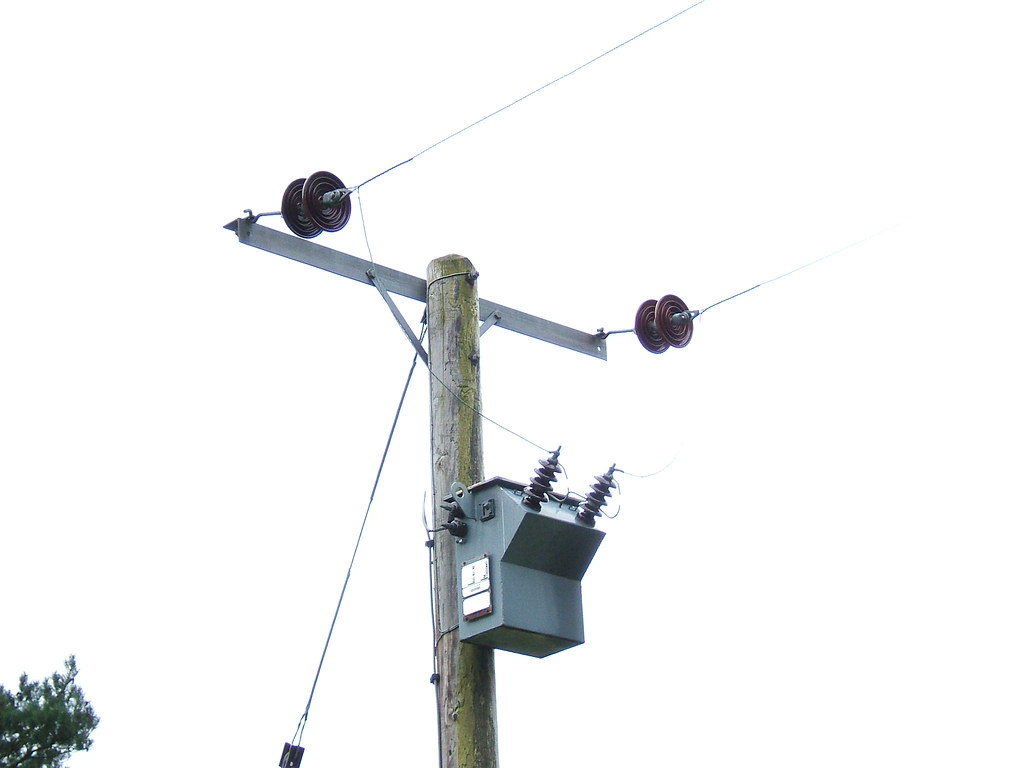# Voltage Drop For Single Phase: How to Calculate And Detailed Facts

This article illustrates Voltage Drop For Single Phase, its calculation, and some relevant questions. Single-phase two-wire systems use a single-phase supply, and a single AC/DC is supplied through one wire.

Voltage drop for a single-phase AC electrical line depends on several factors – length of the line, conductive resistance of the line, phase angle, and the current flowing through the line or the load current. The current and impedance account for the voltage drop between two ends of the single-phase line.

## How To Calculate Voltage Drop For Single Phase?

By single phase, we can think of both AC and DC. While AC supply can be single-phase as well as multi-phase, DC supply is always a single phase. AC and DC single phases have different voltage drop calculations.

We calculate voltage drop in a DC by multiplying the resistance with two times length × current. For single-phase AC connection, we multiply the sine and cosine of the phase angle respectively with the reactance and resistance of the wire, add them and multiply the quantity with two times length × current.

## What is Voltage Drop For Single Phase?

The Voltage Drop For Single Phase is the current multiplied by the impedance of the connection. According to the National Electrical Code, the maximum overall voltage drop of 5% is acceptable for standard efficiency.

A single-phase connection consists of two wires- one phase and one neutral. While the current passes from source to load through the phase wire, the neutral wire arranges for a return path for the current so that everything becomes balanced. The Voltage Drop For Single Phase occurs when current starts flowing.“Electric pole with single phase transformer” by LHOON. Image credit: Flickr

## Single phase voltage drop formula

The Voltage Drop For Single Phase formulas for DC and AC are-

[Latex]V_{DC} = \frac {2\times I\times L\times R} {1000}[/Latex]

[Latex]V_{1\phi } = \frac {2\times I \left ( R cos\theta + X sin\theta \right )\times L } {1000}[/Latex]

Where I= load current, R = wire resistance, X= wire impedance, L= wire length and θ = phase angle( for AC)

We can find the percentage voltage drop by dividing it with supply voltage and multiplying that by 100. The reason we divide the formulas by 1,000 is that typical impedance values are given for every 1,000 feet. This way, we convert them to ohms per foot. Also, we multiply the length by 2 to take the two-way wire length.

## 3 phase voltage drop calculation formula

A three-phase system is always powered by an AC supply. Unlike single-phase, three-phase power uses four wires, out of which three are conducting wires, and one is neutral.

Voltage drop for a three-phase system is-

[Latex]V_{3\phi } = \frac { \sqrt{3} \times I \left ( R cos \theta + X sin \theta \right )\times L } {1000}[/Latex], where I= load current, R = wire resistance, X= wire impedance, L= wire length and θ = phase angle. The √3 quantity in this formula comes from the ratio of the phase-to-phase and phase-to-neutral voltages in a 3-phase connection.

Three phase system; “Fortis Alberta 25kV – Lethbridge County, AB” by Tonyglen14 is licensed under CC BY 2.0

## A single-phase load is connected to a 230 V two-wire single-phase source. The load is located 200 feet from the source. The 230 v source and the load are connected by a two-wire cable. Each conductor has an impedance of (3+0.2j) per 1000 ft. The line current is 8 A. What is the percentage of voltage drop and voltage at the load terminal?

The simple line drawing of the set-up is shown below:

Due to the impedance R= 3+j0.2 ohm and I= 8 A, there will be a voltage drop at the end of the line i.e. on the load side. Let us call the voltage drop as Vd and load voltage as VL.

So, Voltage drop Vd= Source voltage- load voltage=  VS– VL, so VL= VS– Vd

VS= 230 V

We know, voltage drop for single phase = [Latex] \frac { I \times L \times R } {1000} [/Latex]

Therefore, [Latex] V_{d} = \frac{ 8\times 200 \times \left ( 3+ j0.2 \right )} {1000} = 4.8 + j0.32\: V [/Latex]

Vd= 4.8 V

We need to multiply the voltage vd by two as the current flows from the neutral wire as well.

So, the percentage voltage drop [Latex]= \frac{4.8 \times 2} {230} \times 100 = 4.17\: % [/Latex]

And the terminal voltage = (230- 9.6 ) = 220.4 V

Kaushikee Banerjee

I am an electronics enthusiast and currently devoted towards the field of Electronics and Communications . My interest lies in exploring the cutting edge technologies. I'm an enthusiastic learner and I tinker around with open-source electronics. LinkedIn ID- https://www.linkedin.com/in/kaushikee-banerjee-538321175